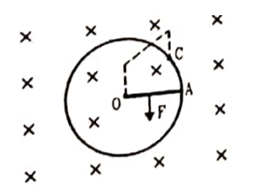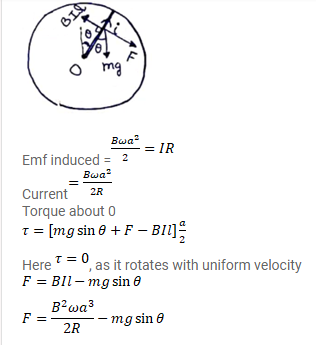# Consider a variation of the previous problem.

Question:

Consider a variation of the previous problem. Suppose the circular loop lies in a vertical plane. The rod has mass $m$. The rod and the loop have negligible resistances but the wire connecting $\mathrm{O}$ and $\mathrm{C}$ has a resistance $\mathrm{R}$. The rod is to made to rotate with a uniform angular velocity $\omega$ in the clockwise direction by applying a force at the midpoint of OA in a direction perpendicular to it. Find the magnitude of this force when the rod makes an angle $\theta$ with the vertical.Solution: